# Gauss–Seidel method

This is to take Jacobi’s Method one step further. Where the better solution is x = (x1, x2, … , xn), if x1(k+1) is a better approximation to the value of x1 than x1(k) is, then it would better that we have found the new value x1(k+1) to use it (rather than the old value that isx1(k)) in finding x2(k+1), … , xn(k+1). So x1(k+1) is found as in Jacobi’s Method, but in finding x2(k+1), instead of using the old value of x1(k) and old values of x3(k),…, xn(k), we then use the new value x1(k+1) and the old values x3(k), … , xn(k), and similarly for finding x3(k+1), … , xn(k+1). This process to find the solution of the given linear equation is called the Gauss-Seidel Method

The Gauss–Seidel method is an iterative technique for solving a square system of n (n=3) linear equations with unknown x.
Given

`Ax=B`

, to find the system of equation x which satisfy this condition.
In more detail, A, x and b in their components are :Then the decomposition of A Matrix into its lower triangular component and its upper triangular component is given by:The system of linear equations are rewritten as: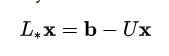The Gauss–Seidel method now solves the left hand side of this expression for x, using previous value for x on the right hand side. More formally, this may be written as: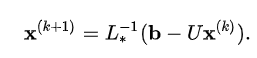However, by triangular form of L*, the elements of x(k+1) can be computed sequentially using forward substitution:This process is continuously repeated until we found the better approximated solution with least error.
Examples:

```Input :
3
4x+ y+ 2z= 4
3x+ 5y+ 1z= 7
x+ y+ 3z= 3

Output :
[0, 0, 0]
[1.0, 0.8, 0.39999999999999997]
[0.6000000000000001, 0.9599999999999997, 0.48000000000000004]
[0.52, 0.9919999999999998, 0.49600000000000005]
[0.504, 0.9983999999999998, 0.4992000000000001]
[0.5008, 0.99968, 0.49984]
[0.5001599999999999, 0.9999360000000002, 0.4999679999999999]
[0.500032, 0.9999872, 0.4999936]
[0.5000064, 0.9999974400000001, 0.49999871999999995]
[0.50000128, 0.999999488, 0.4999997439999999]
[0.500000256, 0.9999998976000001, 0.49999994880000004]
[0.5000000512, 0.9999999795199999, 0.4999999897600001]
[0.50000001024, 0.999999995904, 0.499999997952]
[0.500000002048, 0.9999999991808, 0.49999999959040003]
[0.5000000004095999, 0.9999999998361601, 0.49999999991808003]
[0.50000000008192, 0.9999999999672321, 0.49999999998361594]
[0.500000000016384, 0.9999999999934465, 0.49999999999672307]
[0.5000000000032768, 0.9999999999986894, 0.4999999999993445]
[0.5000000000006554, 0.9999999999997378, 0.49999999999986894]
[0.500000000000131, 0.9999999999999478, 0.49999999999997374]
[0.5000000000000262, 0.9999999999999897, 0.49999999999999467]
[0.5000000000000052, 0.9999999999999979, 0.49999999999999895]
[0.5000000000000011, 0.9999999999999994, 0.49999999999999983]
[0.5000000000000002, 0.9999999999999998, 0.5000000000000001]
[0.49999999999999994, 1.0, 0.5]
[0.5, 1.0, 0.5]
```

Given the three equation:

```4x + y + 2z = 4
3x + 5y + z = 7
x + y + 3z = 3
```

First we assume that the solution of given equation is

`(0,0,0)`

Then first we put value of y and z in equation 1 and get value of x and update the value of x as

`(x1,0,0)`

Now, putting the updated value of x that is x1 and z=0 in equation 2 to get y1 and then updating our solution as

`(x1,y1,0)`

Then, at last putting x1 and y1 in equation 3 to get z1 and updating our solution as

`(x1,y1,z1)`

Now repeat the same process 24 more times to get the approximate solution with minimum error.

 `# Defining our function as seidel which takes 3 arguments ` `# as A matrix, Solution and B matrix ` `  `  `def` `seidel(a, x ,b): ` `    ``#Finding length of a(3)        ` `    ``n ``=` `len``(a)                    ` `    ``# for loop for 3 times as to calculate x, y , z ` `    ``for` `j ``in` `range``(``0``, n):         ` `        ``# temp variable d to store b[j] ` `        ``d ``=` `b[j]                   ` `         `  `        ``# to calculate respective xi, yi, zi ` `        ``for` `i ``in` `range``(``0``, n):      ` `            ``if``(j !``=` `i): ` `                ``d``-``=``a[j][i] ``*` `x[i] ` `        ``# updating the value of our solution         ` `        ``x[j] ``=` `d ``/` `a[j][j] ` `    ``# returning our updated solution            ` `    ``return` `x     ` `  `  `# int(input())input as number of variable to be solved                 ` `n ``=` `3`                               `a ``=` `[]                             ` `b ``=` `[]         ` `# initial solution depending on n(here n=3)                      ` `x ``=` `[``0``, ``0``, ``0``]                         ` `a ``=` `[[``4``, ``1``, ``2``],[``3``, ``5``, ``1``],[``1``, ``1``, ``3``]] ` `b ``=` `[``4``,``7``,``3``] ` `print``(x) ` ` `  `#loop run for m times depending on m the error value ` `for` `i ``in` `range``(``0``, ``25``):             ` `    ``x ``=` `seidel(a, x, b) ` `    ``#print each time the updated solution ` `    ``print``(x)                      `

An example for the matrix version
A linear system shown as Ax=b is given by: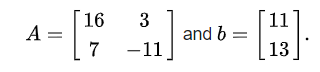We want to use the equation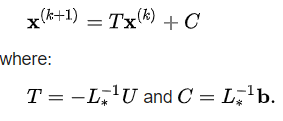Where: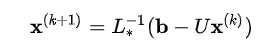We must decompose A into the sum of a lower triangular component L* and a strict upper triangular component U: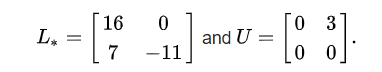The Inverse of L* is:Now we can find remaining things: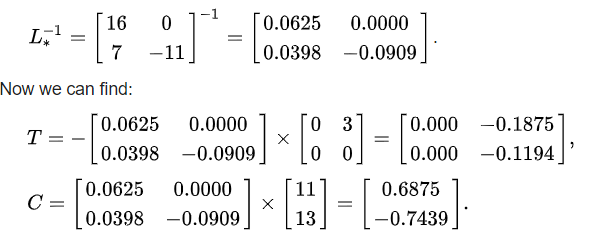Now we have T and C and we can use them to obtain the vectors x iteratively.

First of all, we have to choose x{0} we can only guess. The better the guess, the quicker the algorithm will perform.

We suppose: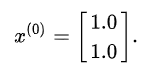Then we can iteratively calculate other x{i’s}: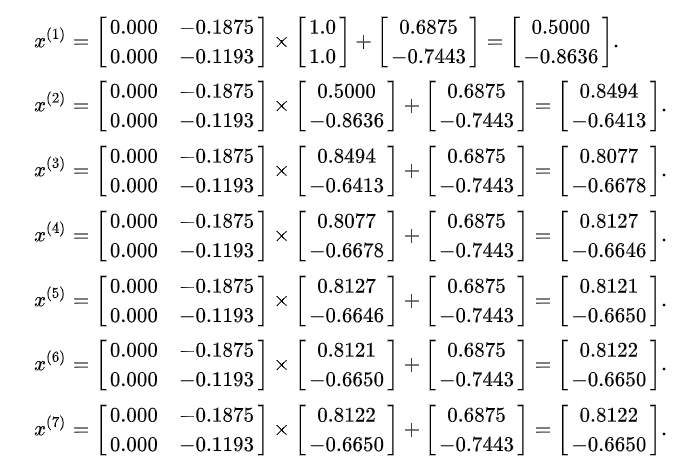Now we know the Exact solution which matches the answer calculated above.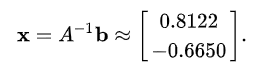In fact, the matrix A is strictly diagonally dominant (but not positive definite).

My Personal Notes arrow_drop_upCheck out this Author's contributed articles.

If you like GeeksforGeeks and would like to contribute, you can also write an article using contribute.geeksforgeeks.org or mail your article to contribute@geeksforgeeks.org. See your article appearing on the GeeksforGeeks main page and help other Geeks.

Please Improve this article if you find anything incorrect by clicking on the "Improve Article" button below.

Article Tags :

Be the First to upvote.

Please write to us at contribute@geeksforgeeks.org to report any issue with the above content.